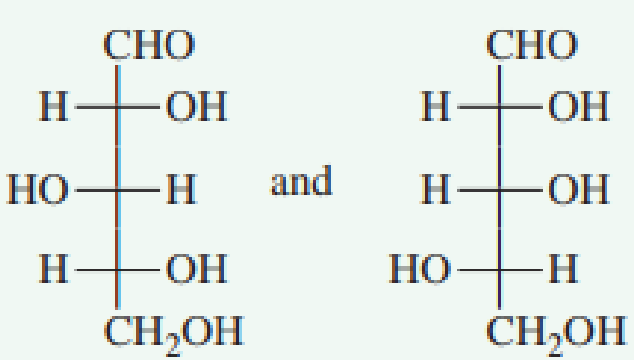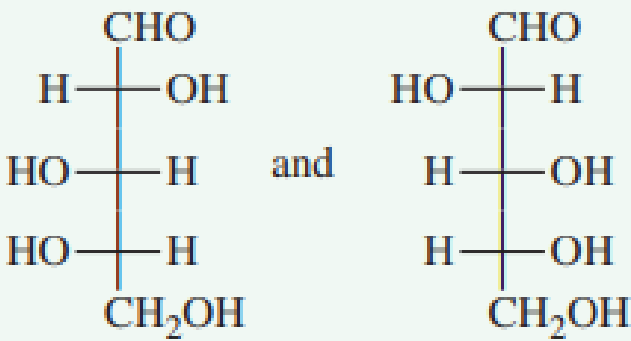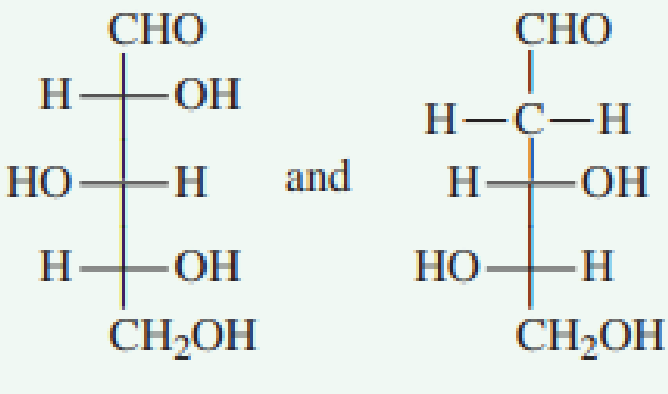Chapter 7.6, Problem 4QQ### Organic And Biological Chemistry

7th Edition
STOKER + 1 other
ISBN: 9781305081079

#### Solutions

Chapter
Section### Organic And Biological Chemistry

7th Edition
STOKER + 1 other
ISBN: 9781305081079
Textbook Problem

# In which pair of Fischer projection formulas are the two monosaccharides diastereomers?a. no correct response

Interpretation Introduction

Interpretation: The pair of Fisher projection formula which represents two monosaccharides diastereomers has to be predicted.

Concept introduction: The two dimensional structural notation which represents the spatial arrangement of group around the chiral centers in molecules is known as the Fisher projection formula.  The chiral centre is indicated by the intersection of horizontal and vertical lines.  The stereoisomers which are not mirror images of each other are known as diastereomers.

Explanation

Reason for correct option:

The pair of Fisher projection formulas in option (a) is shown below.

These pairs of Fisher projection formulas are not mirror images of each other.  These two structures are diastereomers.  All the chiral centers have both an H and OH attachment.  However, a mirror-image relationship between these two attachments is present at only two of the three chiral centers.  At the third chiral center, carbon 2, the H and OH orientation is same

### Still sussing out bartleby?

Check out a sample textbook solution.

See a sample solution

#### The Solution to Your Study Problems

Bartleby provides explanations to thousands of textbook problems written by our experts, many with advanced degrees!

Get Started